# Applications of Derivatives in AP Calculus: Help and Review Chapter Exam

Exam Instructions:

Choose your answers to the questions and click 'Next' to see the next set of questions. You can skip questions if you would like and come back to them later with the yellow "Go To First Skipped Question" button. When you have completed the practice exam, a green submit button will appear. Click it to see your results. Good luck!

### Page 1

#### Question 1 1. Evaluate f(2.2) by linearizing f(x) around x=2.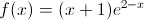#### Question 2 2. You would like to use Newton's method to find the root of f(x). What is the equation you will need to solve?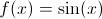### Page 2

#### Question 8 8. Evaluate f(0.1) by linearizing f(x) around x=0.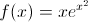### Page 3

#### Question 11 11. Linearize f(x) about the point x=pi/2.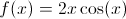#### Question 14 14. Evaluate f(1.1) by linearizing f(x) around x=1.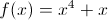### Page 4

#### Question 18 18. Linearize f(x) about the point x=0: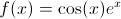#### Question 19 19. You are standing on a building on Mars with a ball, and you have decided that the appropriate equation of motion for the height of the ball as a function of time (t) is below. Which graph represents this equation?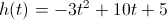### Page 5

#### Question 21 21. You would like to use Newton's method to find the root of f(x). What is the equation you will need to solve?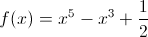#### Question 22 22. You are standing on a building on Jupiter with a ball, and you have decided that the appropriate equation of motion for the height of the ball in miles as a function of (t) is shown below on the graph. What is the highest that the ball gets?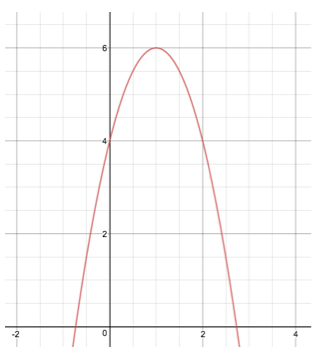#### Question 23 23. Evaluate f(1.1) by linearizing f(x) around x=1.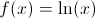#### Question 24 24. Use Newton's method to find the root of f(x), starting at x=1.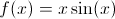#### Question 25 25. Evaluate f(pi + 0.1) by linearizing f(x) around x=pi.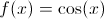### Page 6

#### Question 26 26. Use Newton's method to find the root of f(x), starting at x=2.#### Question 29 29. Linearize f(x) about the point x: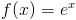#### Question 30 30. Linearize f(x) about the point x=0:#### Applications of Derivatives in AP Calculus: Help and Review Chapter Exam Instructions

Choose your answers to the questions and click 'Next' to see the next set of questions. You can skip questions if you would like and come back to them later with the yellow "Go To First Skipped Question" button. When you have completed the practice exam, a green submit button will appear. Click it to see your results. Good luck!

Support